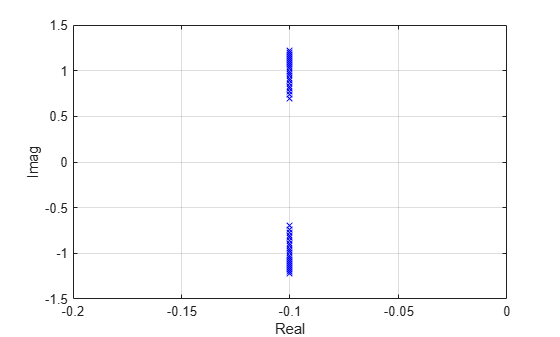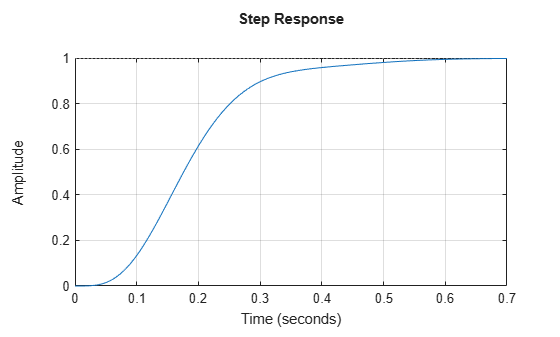# Gain-Scheduled LQG Controller

This example illustrates the loss of stablity that can arise in gain-scheduled control when the operating point changes too quickly. This corresponds to the example on page 102 of . Additional details are given in Example 5.2.1. of .

### LPV Plant

The LPV plant is a second-order model that depends on the parameter $\mathit{p}$. The system has two lightly damped poles for frozen (constant) values of $\mathit{p}$. The plant equations are

`$\left(\begin{array}{c}{\stackrel{˙}{\mathit{x}}}_{1}\\ {\stackrel{˙}{\mathit{x}}}_{2}\end{array}\right)=\left(\begin{array}{cc}0& 1\\ -1-\frac{\mathit{p}}{2}& -0.2\end{array}\right)\left(\begin{array}{c}{\mathit{x}}_{1}\\ {\mathit{x}}_{2}\end{array}\right)+\left(\begin{array}{c}0\\ 1\end{array}\right)\mathit{u}$`

`$\mathit{y}=\left(\begin{array}{cc}1& 0\end{array}\right)\left(\begin{array}{c}{\mathit{x}}_{1}\\ {\mathit{x}}_{2}\end{array}\right)$`

where the parameter $\mathit{p}$ satisfies $|\mathit{p}|\le 1$.

`G = lpvss('p',@GFCN);`

Display the poles at "frozen" parameter values.

```pval = -1:0.1:1; Gs = sample(G,0,pval); Gpole = pole(Gs); Gpole = Gpole(:); plot(real(Gpole),imag(Gpole),'bx'); axis([-0.2 0 -1.5 1.5]); xlabel('Real'); ylabel('Imag') grid on;```### Gain-Scheduled LQG Controller with Integral Action

A gain-scheduled linear-quadratic-Gaussian (LQG) controller combines state feedback with a state observer and includes integral action. The observer and state feedback gains are designed using loop-transfer recovery. The scheduled controller (including integral action) corresponds to ${\mathit{K}}_{\mathit{a}}$ in Figure 1 of .

Augment the plant with an integrator at the input as shown in Figure 1 of . The integrator is implemented with the controller. The integral action ensures good low frequency tracking and perfect rejection of constant disturbances.

`Gaug = G*tf(1,[1 0]);`

Construct the LPV controller using the function `KFCN`. For any parameter value, this function computes LQR and Kalman gains for the corresponding plant matrices.

`Ka = lpvss('p', @(t,p) KFCN(t,p,Gaug));`

### LTI Analysis

Plot the open-loop response for frozen parameter values. Note that the loops are the same at all parameter values. The scheduled controller cancels the lightly damped poles at frozen parameter values.

```L = G*Ka; Ls = sample(L,[],pval); bode(Ls) grid on;```The step responses for frozen parameter values show zero steady-state error, no overshoot, and a settling time of less than one second.

```Ts = feedback(Ls,1); step(Ts) grid on;```### LPV Analysis

Use `feedback` to construct a closed-loop LPV model and plot the LPV step response for slowly-varying parameter $\mathit{p}\left(\mathit{t}\right)$. The response is similar to the LTI responses for frozen $\mathit{p}$.

```T = feedback(L,1); % Slow parameter variation t = linspace(0,10,1000); pt = cos(0.02*t); step(T,t,pt) title('Step response with p(t)=cos(0.02t)') grid on```Next consider fast parameter variations, $\mathit{p}\left(\mathit{t}\right)=\mathrm{cos}\left(2\mathit{t}\right)$. The step response now shows instability.

```% Fast parameter variation t = linspace(0,100,1000); pt = cos(2*t); step(T,t,pt); title('Step response with p(t)=cos(2t)') grid on```This plot corresponds to Figure 2 in . The instability here results from the frozen parameters not reflecting the true dynamics. For more information, see "Potential Hazards of Gain Scheduling" in .

### Plant and Controller Data Functions

`type GFCN`
```function [A,B,C,D,E,dx0,x0,u0,y0,Delays] = GFCN(~,p) % Clip p to range [-1,1] p = max(-1,min(p,1)); A = [0 1; -1-p/2 -0.2]; B = [0; 1]; C = [1 0]; D = 0; E = []; dx0 = []; x0 = []; u0 = []; y0 = []; Delays = []; ```
`type KFCN`
```function [A,B,C,D,E,dx0,x0,u0,y0,Delays] = KFCN(t,p,Gaug) % This code solves Riccati equations and forms the gains for each value % of p. This is not particularly efficient especially if used for % simulations or real-time implementation. An alternative would be to % pre-solve either the Riccati equations (or the gains) on a grid of % parameters and then interpolate. % Sample augmented plant to get dynamics at (t,p) [Aaug,Baug,Caug,~] = ssdata(sample(Gaug,t,p)); % Design Parameters N = [1; 0; 1+p/2]; mu = 0.01; rho = 1e-8; % Solve observer and state-feedback Riccati equations % The observer and state-feedback gains are given by: % H = Sigma*(Caug)'/mu and G = (Baug)'*Z/rho; [~,Ht] = icare(Aaug',Caug',N*N',mu); H = Ht'; [~,G] = icare(Aaug,Baug,Caug'*Caug,rho); % Observer / state-feedback with integral action (See Fig. 1 of ). K = ss(Aaug-Baug*G-H*Caug, -H,-G,0); Ka = tf(1,[1 0])*K; [Ak,Bk,Ck,Dk] = ssdata(Ka); % State-space and offset data: A = Ak; B = Bk; C = Ck; D = Dk; E = []; dx0 = []; x0 = []; u0 = []; y0 = []; Delays = []; ```

### References

 Shamma, J. S., and M. Athans. “Gain Scheduling: Potential Hazards and Possible Remedies.” IEEE Control Systems 12, no. 3 (June 1992): 101–7. https://doi.org/10.1109/37.165527.

 Shamma, "Analysis and Design of Gain Scheduled Control Systems," Ph.D. Thesis, MIT, 1988.